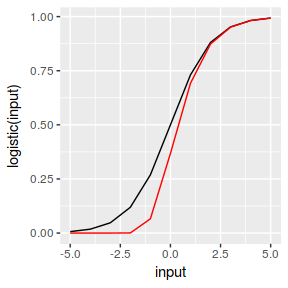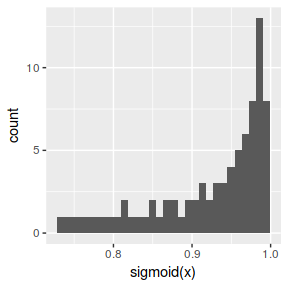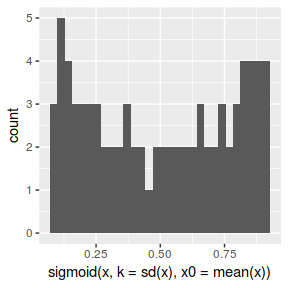# sigmoid

#### 2018-06-21

The sigmoid() function returns the sigmoid value of the input(s), by default this is done using the standard logistic function.

library(sigmoid)
sigmoid(3)
##  0.9525741

Inputs can also be tensors, such as vectors, matrices, or arrays.

sigmoid(-5:5)
##   0.006692851 0.017986210 0.047425873 0.119202922 0.268941421
##   0.500000000 0.731058579 0.880797078 0.952574127 0.982013790
##  0.993307149
sigmoid( matrix(-3:5,nrow=3) ) # etc.
##            [,1]      [,2]      [,3]
## [1,] 0.04742587 0.5000000 0.9525741
## [2,] 0.11920292 0.7310586 0.9820138
## [3,] 0.26894142 0.8807971 0.9933071

The sigmoid() function is a wrapper, which by default uses the logistic() function, it can also use other methods.

## Gompertz

sigmoid( -5:5, method='Gompertz' )
##   3.507389e-65 1.942338e-24 1.892179e-09 6.179790e-04 6.598804e-02
##   3.678794e-01 6.922006e-01 8.734230e-01 9.514320e-01 9.818511e-01
##  9.932847e-01

These functions can also be accessed directly.

Gompertz(-1:-5)
##  6.598804e-02 6.179790e-04 1.892179e-09 1.942338e-24 3.507389e-65

## Rectified Linear Unit (ReLU)

Rectified Linear Unit (ReLU)

sigmoid( -5:5, method='ReLU')
##   0 0 0 0 0 0 1 2 3 4 5

Leaky Rectified Linear Unit

sigmoid( -5:5, method="leakyReLU")
##   -0.05 -0.04 -0.03 -0.02 -0.01  0.00  1.00  2.00  3.00  4.00  5.00

## Mappings

These mappings are similar but not identical.

library(ggplot2)

input = -5:5

df = data.frame(input, logistic(input), Gompertz(input))

ggplot(df, aes(input, logistic(input))) + geom_line() +
geom_line(aes(input,Gompertz(input)), colour='red')## Inverse

The wrapper can also apply the inverse of the method, returning the original values.

sigmoid( sigmoid(-5:5), inverse=TRUE )
##   -5 -4 -3 -2 -1  0  1  2  3  4  5

Which also works for other methods.

sigmoid( sigmoid(-5:5, method='Gompertz'), method='Gompertz', inverse=TRUE )
##   -5 -4 -3 -2 -1  0  1  2  3  4  5

In addition to this, the SoftMax algorithm can be pre applied.

sigmoid( -3:5 )
sigmoid( -3:5, SoftMax = TRUE )
##  0.04742587 0.11920292 0.26894142 0.50000000 0.73105858 0.88079708
##  0.95257413 0.98201379 0.99330715
##  0.01006486 0.03102509 0.09159656 0.24101050 0.50000000 0.75898950
##  0.90840344 0.96897491 0.98993514

Several parameters can be specified (for details see help(logistic), etc.). This can for instance be used to preserve greater entropy.

x = seq(1,5, by=0.05)
qplot(sigmoid(x))qplot( sigmoid(x, k=sd(x), x0=mean(x) ) )